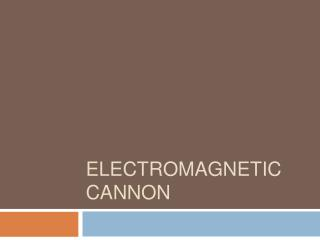DownloadDownload PresentationELECTROMAGNETIC CANNON

# ELECTROMAGNETIC CANNON

Download Presentation## ELECTROMAGNETIC CANNON

- - - - - - - - - - - - - - - - - - - - - - - - - - - E N D - - - - - - - - - - - - - - - - - - - - - - - - - - -
##### Presentation Transcript

1. ELECTROMAGNETIC CANNON

2. Question • A solenoid can be used to fire a small ball. A capacitor is used to energize the solenoid coil. Build a device with a capacitor charged to a maximum 50V. • Investigate the relevant parameters and maximize the speed of the ball.

3. Outline • Experimental setup • Experimental procedure • Typical result • Statistics and explanation • Relative parameters

4. Experimental Setup

5. Experimental Procedure • Charge the capacitor five times longer than its time constant. • Connect the capacitor to the solenoid. • Measure the distance the bar flies to estimate the initial speed. • Repeat the procedure above.

6. Video Typical Result

7. Qualitative Explanation • The “steel” ball is magnetized, with the same direction as the magnetic field in the solenoid. • Faraday’s Law • Curie’s Law

8. Why It Moves • A magnetic dipole in magnetic field tends to move toward where the magnetic potential is less (i.e. the middle of the solenoid)‏ • Magnetic domain model • Loop model

9. How Fast It Moves • The magnetic field is associated with the discharging process of the capacitor, and the position of the ball. • The dipole moment is associated with the changing rate of the magnetic field, and the magnetization of the ball.

10. Parameters • Voltage • Solenoid • Length • Layer • Diameter (→Inductance) • Capacitance • Resistance (→frequency)‏ • Magnetic field • Ball • Magnetic permeability • Resistance • Mass • Shape • Initial position

11. Experiment I • Speed vs. Voltage • Parameter : Voltage (from 30V ~50V)‏ • Initial Condition : • Capacitance: 1470μF • Number of Layers: 5 layers • Length: 1.5 cm • Position of Cannon: In front of the solenoid (0 mm)‏ • Mass: 0.014 g

12. Voltage • Speed is positively related to the voltage.

13. Experiment II • Speed vs. Capacitance • Parameter : Capacitance (from 470μF to 3300μF)‏ • Initial Condition : • Voltage: 50 V • Number of Layers: 5 layers • Length: 1.5 cm • Position of Cannon: In front of the solenoid (0 mm)‏ • Mass: 0.014 g

14. Capacitance • A peak occurs at 1470 μF.

15. 1.5 cm 6 8 7 4 5 3 2 1 Experiment III • Speed vs. Number of Layers • Parameter : Number of layers (4~8 layers)‏ • Initial Condition : • Voltage: 50 V • Capacitance: 1470 μF • Length: 1.5 cm • Position of Cannon: In front of the solenoid (0 mm)‏ • Mass: 0.014 g

16. Numbers of Layers • A peak occurs at 5 layers.

17. Experiment IV • Speed vs. Solenoid length • Parameter : Length (1, 1.5, 2, 2.5, 3 cm)‏ • Initial Condition : • Voltage: 50 V • Capacitance: 1470 μF • Number of Layers: 5 cm • Position of Cannon: In front of the solenoid (0 mm)‏ • Mass: 0.014 g

18. Length • A peak occurs at 1.5 cm.

19. Experiment V • Speed vs. Position • Parameter : Position (0~10 mm)‏ • Initial Condition : • Voltage: 50 V • Capacitance: 3700 μF • Length: 1.0 cm • Mass: 0.083 g

20. Position • Speed reaches maximum when the ball is at the front of the solenoid.

21. Summary • The fastest speed we achieve is 31.4 m/s, which happens under • V=50 V • C=1470 μF • Layers=5 • Solenoid Length=1.5 cm • Position: At the front • Ball mass=0.014g • Material: Iron

22. Summary • In this case, energy stored in the capacitor is 1.84 J. • Energy transferred to the projectile is 6.91*10-3 J. • Ratio=3.76*10-3

23. Thank you!